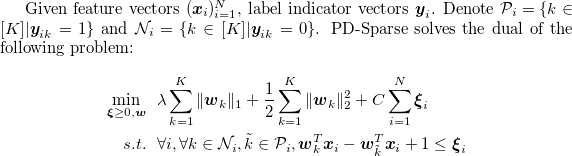# PD-Sparse -- A Primal and Dual Sparse Approach to Extreme Multiclass and Multilabel Classification

## Description

PD-Sparse is a solver for Extreme Multiclass/Multilabel problem of 103~106 or more classes. PD-Sparse employs a (Dual) Fully-Corrective Block-Coordinate Frank-Wolfe algorithm that exploits both primal and dual sparsity to achieve a complexity sublinear to the number of primal and dual variables, which leads to prediction and training time not growing linear with the number of classes.## Download code and Compile

### You can download the code from [code] (updated on May 23, 2017) , or by

```\$ git clone https://github.com/a061105/ExtremeMulticlass.git
```

### Compile it by "make" in a unix based system with support of g++ of version 4.7+.

Three binary files will be generated: multiTrain , multiTrainHash and multiPred. Both multiTrain and multiTrainHash can be used for training since they solve the exact same problem. Note that multiTrainHash is designed to be more memory-efficient whereas multiTrain is faster when memory is sufficient (i.e. when matrices of size (#classes by #features) can fit into memory).

## Downloading data:

```We provide the (train, heldout and test) data sets used in our paper.
The data sets are listed below:

multilabel data sets:
"Eur-Lex", "rcv1_regions", "bibtex", "LSHTCwiki"

multiclass data sets:
"sector", "aloi.bin", "Dmoz", "LSHTC1", "imageNet"

Note the data sets can be also found on other public sites (e.g. libsvm, mulan dataset pages and LSHTC competition sites), but the versions here have been preprocessed (e.g. tf-idf, random features, and scaling).

One can download them by

\$ cd examples/
\$ make construct dataset=rcv1_regions

Note that the exact names of data sets listed above should be assigned to variable "dataset".
```

## Training:

```One can train by either of the two commands:

\$ ./multiTrain (options) [train_data] (model)
\$ ./multiTrainHash (options) [train_data] (model)
options::
-s solver: (default 1)
0 -- Stochastic Block Coordinate Descent
1 -- Stochastic-Active Block Coordinate Descent (PD-Sparse)
-l lambda: L1 regularization weight (default 0.1)
-c cost: cost of each sample (default 1.0)
-r speed_up_rate: sample 1/r fraction of non-zero features to estimate gradient (default r = ceil(min( 5DK/(Clog(K)nnz(X)), nnz(X)/(5N) )) )
-q split_up_rate: divide all classes into q disjoint subsets (default 1)
-m max_iter: maximum number of iterations allowed if -h not used (default 50)
-u uniform_sampling: use uniform sampling instead of importance sampling (default not)
-g max_select: maximum number of dual variables selected during search (default: -1 (i.e. dynamically adjusted during iterations) )
-p post_train_iter: #iter of post-training without L1R (default auto)
-h <file>: using accuracy on heldout file '<file>' to terminate iterations
-e early_terminate: how many iterations of non-increasing heldout accuracy required to earyly stop (default 3)
```

#### Examples:

```Train models on data sets "rcv1_regions" and "sector":

\$ ./multiTrain -h ./examples/rcv1_regions/rcv1_regions.heldout ./examples/rcv1_regions/rcv1_regions.train rcv1_regions.model
\$ ./multiTrain -h ./examples/sector/sector/sector.heldout ./examples/sector/sector/sector.train sector.model

Note that models generated by post training will be stored separately with name "rcv1_regions.model.p" and "sector.model.p".
```

## Prediction:

One can use the following command to do prediction:
```\$ ./multiPred [testfile] [model] (-p S <output_file>) (k)
```
this command compute top k accuracy and print top S <label>:<prediction score> pairs to <output_file>, one line for each instance. (default S=0 and no file is generated; k = 1)

#### Examples:

```Compute top-1 accuracy of the model generated:

\$ ./multiPred ./examples/rcv1_regions/rcv1_regions.test rcv1_regions.model 1

Compute test accuracy of the model generated by post training:

\$ ./multiPred ./examples/sector/sector.test sector.model.p 1

Compute (top-1) test accuracy and also print top-5 "label:score" pairs to file "sector.table":

\$ ./multiPred ./examples/sector/sector.test sector.model -p 5 sector.table 1

```

## Run examples with Makefile:

```For data sets used in our paper, we provide a simple script to download, train and test on them automatically. For example, one can run

make rcv1_regions

to get all above things done for all data sets we used.
```

## Input Format:

We use libsvm format: <true label 1>,<true label 2>,...,<true label T>   <index1>:<value1>   <index2>:<value2> ...
.
.
.

#### Examples:

```\$ head -1 ./examples/multiclass/sector/sector.train

53 1:0.00049 2:0.0009 3:4e-05 5:0.00054 6:0.00458 8:0.01302 ... 41303:0.14897

\$ head -1 ./examples/multilabel/rcv1_regions.train

53,112 440:0.046331 730:0.098266 ... 46694:0.062828

```

## Output Format(with examples):

```Our model file format looks like:

nr_class <K(number of classes)>
label <label 1> ... <label K>
nr_features <D(number of features)>
<nnz(w)> <index 1>:<w[index 1]> <index 2>:<w[index 2]> ... <index nnz(w)>:<w[index nnz(w)]>
<nnz(w)> <index 1>:<w[index 1]> <index 2>:<w[index 2]> ... <index nnz(w)>:<w[index nnz(w)]>
.
.
.
<nnz(w[D-1])> ...
```

#### Examples:

```\$ head -5 model

nr_class 228
label 293 ... 171 352
nr_feature 47237
0
6 65:0.223974 3:0.00958934 17:0.0270412 11:0.134858 42:-0.0397849 98:-0.0188011

```

### Citation:

PD-Sparse: A Primal and Dual Sparse Approach to Extreme Multiclass and Multilabel Classification.
Ian E.H. Yen*, Xiangru Huang*, Kai Zhong, Pradeep Ravikumar and Inderjit S. Dhillon. (* equally contributed)
In International Conference on Machine Learning (ICML), 2016. [pdf]

### Suggestion and Bug report:

```For any suggestion and bug report, feel free to contact:
Ian En-Hsu Yen(eyan@cs.cmu.edu), Xiangru Huang(xrhuang@cs.utexas.edu)
```